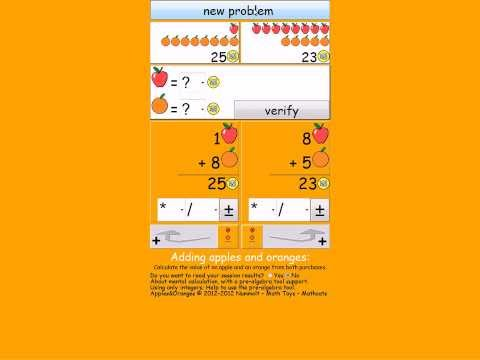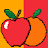1K+Everyone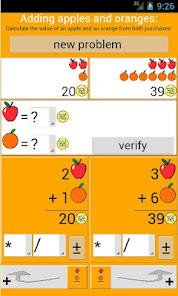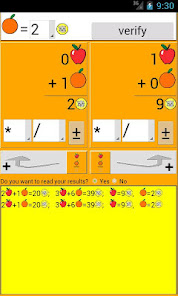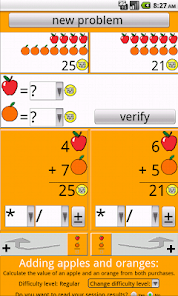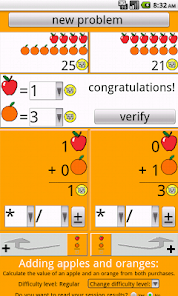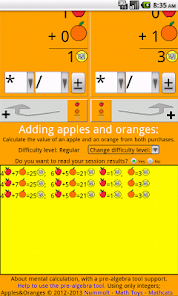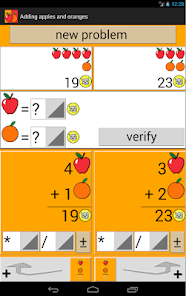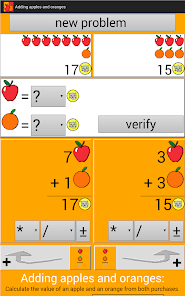To calculate the value of an apple and an orange from 2 purchases.
(Assuming that all apples are equal and that all oranges are equal)
About mental arithmetic with a pre-algebra tool, introducing the Gaussian elimination. ( Gauss - Jordan )
From http://www.nummolt.com/obbl/appleorange/index.html
nummolt - Obbl - Math Toys collection - Mathcats.
Nummolt apps: "Not Montessori per se, but Montessori-like".

MathTools reference:
http://mathforum.org/mathtools/tool/178345/

Algebra Linear Systems
Algebra II Solving systems of equations, Elimination
PreCalculus Solving systems of linear equations, Gauss-Jordan Method, determinants

We recommend the paid 'Apples and Oranges 1 Decimal':
(http://mathforum.org/mathtools/tool/219536/)
Math 7: Linear relationships, Find slope, Slope-intercept form, Equation of the line, Rect coordinate geometry, First quadrant, Geometry in the plane
Algebra: Linear Equations, Slope Between Points, Finding Intercepts, Slope - Intercept Form, Point - Slope Form, Graphing, Parallel Lines, Perpendicular Lines, Linear Formulas, Analyzing Linear Data, Is It Linear?, Writing an Equation, Line of Best Fit, Linear Systems, Solve by graphing, Solve by substitution, Solve by combination, Inconsistent System, Applications
Algebra II: Solving systems of equations, Cramer's rule, Matrices, Elimination, Substitution

NOTE:
'Adding apples and oranges' app free, is a simplified version of the first part of the nummolt's paid app: 'Apples and oranges 1 decimal place'. (the Gauss-Jordan elimination)
All options "Apples and oranges first decimal":
( 1: Gauss-Jordan elimination; 2: Graphic; 3: Substitution; 4: Cramer's rule; 5: Inverse matrix )

Credits:
Feature graphic by: http://photo-lgz.blogspot.com/
Updated on
Oct 1, 2020

## Data safety

Safety starts with understanding how developers collect and share your data. Data privacy and security practices may vary based on your use, region, and age. The developer provided this information and may update it over time.No data shared with third parties